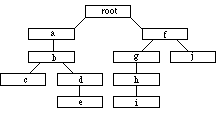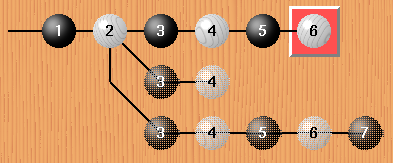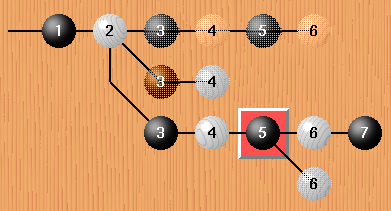last updated: 1999-01-07

# Variations

SGF's internal structure is a tree of property lists. This allows storing variations of the main line of play. The tree is written in pre-order. Here's a short sketch for an algorithm for writing a tree in pre-order:

 Algorithm Example tree pre-order ```WriteTree(Root) End WriteTree(Node) Write(Node) for each child of Node WriteTree(child) end for end ```root a b c d e f g h i j SGF ```(;FFC[root](;C[a];C[b](;C[c]) (;C[d];C[e])) (;C[f](;C[g];C[h];C[i]) (;C[j]))) ```

Have a look at the EBNF definition again. Check the SGF example file and the User Guide for more details, such as problems with annotations and variations. Related properties: ST, C, N.

Here are some examples to make it easier to understand the EBNF definition and its application to variations.
Pictures taken from cgoban

 No Variation`(;FFGMSZ;B[aa];W[bb];B[cc];W[dd];B[ad];W[bd])` One variation at move 3```(;FFGMSZ;B[aa];W[bb](;B[cc];W[dd];B[ad];W[bd]) (;B[hh];W[hg]))``` Note the beginning of a new game tree in front of B[cc] and the variation itself on the second line. There are two ")" at the end: one for the variation gametree and one for the end of the main gametree starting at FF. Two variations at move 3```(;FFGMSZ;B[aa];W[bb](;B[cc]N[Var A];W[dd];B[ad];W[bd]) (;B[hh]N[Var B];W[hg]) (;B[gg]N[Var C];W[gh];B[hh];W[hg];B[kk]))``` Usually the main line is labeled variation "A", the first variation "B", the second variation "C" and so on. That's why many annotations refer to the next move as "A". Two variations at different moves```(;FFGMSZ;B[aa];W[bb](;B[cc];W[dd](;B[ad];W[bd]) (;B[ee];W[ff])) (;B[hh];W[hg]))``` The new gametree starts in front of B[ad]. Note that the second varition (2nd line) is in front of the first variation (3rd line). Have a look at the closing brackets as well. Variation of a variation```(;FFGMSZ;B[aa];W[bb](;B[cc]N[Var A];W[dd];B[ad];W[bd]) (;B[hh]N[Var B];W[hg]) (;B[gg]N[Var C];W[gh];B[hh] (;W[hg]N[Var A];B[kk]) (;W[kl]N[Var B])))``` The new game tree starts in front of W[hg]. Note that there are three ")" at the end: one for the W[kl] variation, one for the B[gg] variation, and one for the main gametree.

## Common pitfalls:

Every variation has at least one node (see EBNF definition)! That is, the smallest possible variation looks like (;) - () is an error! Another example: removing all properties from the "variation of a variation" example leads to: (;;;(;;;;)(;;)(;;;(;;)(;))) and additionally removing unnecessary nodes leads to (;(;)(;)(;(;)(;)))

Properties are part of a node, therefore (W[tt]) is an error. Correct is (;W[tt])

No properties outside a gametree! E.g. (;)W[tt] is an error.